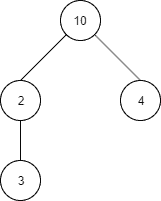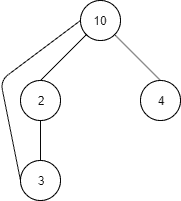## 1. Overview

In this tutorial, we’re going to learn about the Breadth-First Search algorithm, which allows us to search for a node in a tree or a graph by traveling through their nodes breadth-first rather than depth-first.

First, we’ll go through a bit of theory about this algorithm for trees and graphs. After that, we’ll dive into the implementations of the algorithms in Java. Finally, we’ll cover their time complexity.

The basic approach of the Breadth-First Search (BFS) algorithm is to search for a node into a tree or graph structure by exploring neighbors before children.

First, we’ll see how this algorithm works for trees. After that, we’ll adapt it to graphs, which have the specific constraint of sometimes containing cycles. Finally, we’ll discuss the performance of this algorithm.

### 2.1. Trees

The idea behind the BFS algorithm for trees is to maintain a queue of nodes that will ensure the order of traversal. At the beginning of the algorithm, the queue contains only the root node. We’ll repeat these steps as long as the queue still contains one or more nodes:

• Pop the first node from the queue
• If that node is the one we’re searching for, then the search is over
• Otherwise, add this node’s children to the end of the queue and repeat the steps

Execution termination is ensured by the absence of cycles. We’ll see how to manage cycles in the next section.

### 2.2. Graphs

In the case of graphs, we must think of possible cycles in the structure. If we simply apply the previous algorithm on a graph with a cycle, it’ll loop forever. Therefore, we’ll need to keep a collection of the visited nodes and ensure we don’t visit them twice:

• Pop the first node from the queue
• Check if the node has already been visited, if so skip it
• If that node is the one we’re searching for, then the search is over
• Otherwise, add it to the visited nodes
• Add this node’s children to the queue and repeat these steps

## 3. Implementation in Java

Now that the theory has been covered, let’s get our hands into the code and implement these algorithms in Java!

### 3.1. Trees

First, we’ll implement the tree algorithm. Let’s design our Tree class, which consists of a value and children represented by a list of other Trees:

``````public class Tree<T> {
private T value;
private List<Tree<T>> children;

private Tree(T value) {
this.value = value;
this.children = new ArrayList<>();
}

public static <T> Tree<T> of(T value) {
return new Tree<>(value);
}

Tree<T> newChild = new Tree<>(value);
return newChild;
}
}``````

To avoid creating cycles, children are created by the class itself, based on a given value.

After that, let’s provide a search() method:

``````public static <T> Optional<Tree<T>> search(T value, Tree<T> root) {
//...
}``````

As we mentioned earlier, the BFS algorithm uses a queue to traverse the nodes. First of all, we add our root node to this queue:

``````Queue<Tree<T>> queue = new ArrayDeque<>();

Then, we’ve got to loop while the queue is not empty, and each time we pop out a node from the queue:

``````while(!queue.isEmpty()) {
Tree<T> currentNode = queue.remove();
}``````

If that node is the one we’re searching for, we return it, else we add its children to the queue:

``````if (currentNode.getValue().equals(value)) {
return Optional.of(currentNode);
} else {
}``````

Finally, if we visited all the nodes without finding the one we’re searching for, we return an empty result:

``return Optional.empty();``

Let’s now imagine an example tree structure:Which translates into the Java code:

``````Tree<Integer> root = Tree.of(10);

Then, if searching for the value 4, we expect the algorithm to traverse nodes with values 10, 2 and 4, in that order:

``BreadthFirstSearchAlgorithm.search(4, root)``

We can verify that with logging the value of the visited nodes:

``````[main] DEBUG  c.b.a.b.BreadthFirstSearchAlgorithm - Visited node with value: 10
[main] DEBUG  c.b.a.b.BreadthFirstSearchAlgorithm - Visited node with value: 2
[main] DEBUG  c.b.a.b.BreadthFirstSearchAlgorithm - Visited node with value: 4``````

### 3.2. Graphs

That concludes the case of trees. Let’s now see how to deal with graphs. Contrarily to trees, graphs can contain cycles. That means, as we’ve seen in the previous section, we have to remember the nodes we visited to avoid an infinite loop. We’ll see in a moment how to update the algorithm to consider this problem, but first, let’s define our graph structure:

``````public class Node<T> {
private T value;
private Set<Node<T>> neighbors;

public Node(T value) {
this.value = value;
this.neighbors = new HashSet<>();
}

public void connect(Node<T> node) {
if (this == node) throw new IllegalArgumentException("Can't connect node to itself");
}
}``````

Now, we can see that, in opposition to trees, we can freely connect a node with another one, giving us the possibility to create cycles. The only exception is that a node can’t connect to itself.

It’s also worth noting that with this representation, there is no root node. This is not a problem, as we also made the connections between nodes bidirectional. That means we’ll be able to search through the graph starting at any node.

First of all, let’s reuse the algorithm from above, adapted to the new structure:

``````public static <T> Optional<Node<T>> search(T value, Node<T> start) {
Queue<Node<T>> queue = new ArrayDeque<>();

Node<T> currentNode;

while (!queue.isEmpty()) {
currentNode = queue.remove();

if (currentNode.getValue().equals(value)) {
return Optional.of(currentNode);
} else {
}
}

return Optional.empty();
}``````

We can’t run the algorithm like this, or any cycle will make it run forever. So, we must add instructions to take care of the already visited nodes:

``````while (!queue.isEmpty()) {
currentNode = queue.remove();
LOGGER.debug("Visited node with value: {}", currentNode.getValue());

if (currentNode.getValue().equals(value)) {
return Optional.of(currentNode);
} else {
}
}

return Optional.empty();``````

As we can see, we first initialize a Set that’ll contain the visited nodes.

``Set<Node<T>> alreadyVisited = new HashSet<>();``

Then, when the comparison of values fails, we add the node to the visited ones:

``alreadyVisited.add(currentNode);``

Finally, after adding the node’s neighbors to the queue, we remove from it the already visited nodes (which is an alternative way of checking the current node’s presence in that set):

``queue.removeAll(alreadyVisited);``

By doing this, we make sure that the algorithm won’t fall into an infinite loop.

Let’s see how it works through an example. First of all, we’ll define a graph, with a cycle:And the same in Java code:

``````Node<Integer> start = new Node<>(10);
Node<Integer> firstNeighbor = new Node<>(2);
start.connect(firstNeighbor);

Node<Integer> firstNeighborNeighbor = new Node<>(3);
firstNeighbor.connect(firstNeighborNeighbor);
firstNeighborNeighbor.connect(start);

Node<Integer> secondNeighbor = new Node<>(4);
start.connect(secondNeighbor);``````

Let’s again say we want to search for the value 4. As there is no root node, we can begin the search with any node we want, and we’ll choose firstNeighborNeighbor:

``BreadthFirstSearchAlgorithm.search(4, firstNeighborNeighbor);``

Again, we’ll add a log to see which nodes are visited, and we expect them to be 3, 2, 10 and 4, only once each in that order:

``````[main] DEBUG c.b.a.b.BreadthFirstSearchAlgorithm - Visited node with value: 3
[main] DEBUG c.b.a.b.BreadthFirstSearchAlgorithm - Visited node with value: 2
[main] DEBUG c.b.a.b.BreadthFirstSearchAlgorithm - Visited node with value: 10
[main] DEBUG c.b.a.b.BreadthFirstSearchAlgorithm - Visited node with value: 4``````

### 3.3. Complexity

Now that we’ve covered both algorithms in Java, let’s talk about their time complexity. We’ll use the Big-O notation to express them.

Let’s start with the tree algorithm. It adds a node to the queue at most once, therefore visiting it at most once also. Thus, if n is the number of nodes in the tree, the time complexity of the algorithm will be O(n).

Now, for the graph algorithm, things are a little bit more complicated. We’ll go through each node at most once, but to do so we’ll make use of operations having linear-complexity such as addAll() and removeAll().

Let’s consider n the number of nodes and c the number of connections of the graph. Then, in the worst case (being no node found), we might use addAll() and removeAll() methods to add and remove nodes up to the number of connections, giving us O(c) complexity for these operations. So, provided that c > n, the complexity of the overall algorithm will be O(c). Otherwise, it’ll be O(n). This is generally noted O(n + c), which can be interpreted as a complexity depending on the greatest number between n and c.

Why didn’t we have this problem for the tree search? Because the number of connections in a tree is bounded by the number of nodes. The number of connections in a tree of n nodes is n – 1.